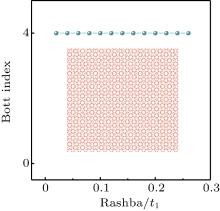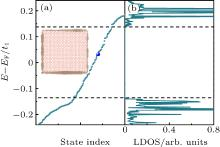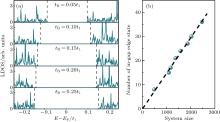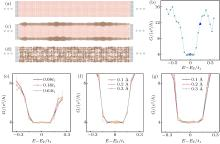Please wait a minute...
 Chin. Phys. B, 2020, Vol. 29(10): 107101    DOI: 10.1088/1674-1056/abab77
 CONDENSED MATTER: ELECTRONIC STRUCTURE, ELECTRICAL, MAGNETIC, AND OPTICAL PROPERTIES Prev   Next

# Quantum anomalous Hall effect in twisted bilayer graphene quasicrystal

Zedong Li(李泽东) and Z F Wang(王征飞)†
1 Hefei National Laboratory for Physical Sciences at the Microscale, CAS Key Laboratory of Strongly-Coupled Quantum Matter Physics, University of Science and Technology of China, Hefei 230026, China
Abstract

The nontrivial topology is investigated in a dodecagonal quasicrystal made of 30° twisted bilayer graphene (TBG). Based on tight-binding model with both exchange field and Rashba spin–orbit coupling, the topological index, chiral edge states, and quantum conductance are calculated to distinguish its unique topological phase. A high Bott index (B = 4) quantum anomalous Hall effect (QAHE) is identified in TBG quasicrystal, which is robust to a finite perturbation without closing the nontrivial gap. Most remarkably, we have found that the multiple Dirac cone replicas in TBG quasicrystal are only a spectra feature without generating extra chiral edge states. Our results not only propose a possible way to realize the QAHE in quasicrystal, but also identify the continuity of nontrivial topology in TBG between crystal and quasicrystal.

Keywords:  quasicrystal      twisted bilayer graphene      quantum anomalous Hall effect
Received:  06 May 2020      Revised:  24 July 2020      Accepted manuscript online:  01 August 2020
 PACS: 71.23.Ft (Quasicrystals) 73.43.Cd (Theory and modeling) 72.15.Cz (Electrical and thermal conduction in amorphous and liquid metals and Alloys ?)
Corresponding Authors:  Corresponding author. E-mail: zfwang15@ustc.edu.cnFig. 1.  Bott index of TBG quasicrystal with different intensities of tR. The inset is the atomic structure of TBG quasicrystal. λ = 0.18t1 and t2 = 0.12t1.Fig. 2.  (a) Discrete energy levels of TBG quasicrystal. (b) Bulk LDOS of TBG quasicrystal. The two dashed lines denote the region of bulk gap. The discrete energy levels in bulk gap are the in-gap chiral edge states. The spatial distribution of in-gap chiral edge state at the energy level marked by the blue dot is shown in the inset of (a), which is localized at the boundary of TBG quasicrystal. The circle size denotes the weight of chiral edge state. λ = 0.18t1, tR = 0.2t1, t2 = 0.12t1.Fig. 3.  (a) Bulk LDOS with different intensities of tR. The two dashed lines denote the region of bulk gap. λ = 0.18t1, t2 = 0.12t1. (b) The number of in-gap edge state vs. the system size. The dashed line is a linear fitting of the calculated results. tR = 0.2t1.Fig. 4.  (a) Device setup of the TBG quasicrystal ribbon. The square lattice denotes the semi-infinite lead region. (b) Conductance vs. the energy without disorder. (c) and (d) LDOS distribution in the center-scattering region at the energy level marked by the blue dots in (b). (e)–(g) Conductance vs. the energy with on-site-energy, interlayer, and intralayer hopping disorder, respectively. The error bar denotes the fluctuation of conductance among different disorder configurations. λ = 0.18t1, tR = 0.2t1, t2 = 0.12t1.